# 正三角形排列管束结构流弹失稳流体力模型数值研究*

(中国核动力研究设计院 核反应堆系统设计技术重点实验室, 成都 610213)

## 1 数值模型建立与验证

### 1.1 管束静止绕流数值模型建立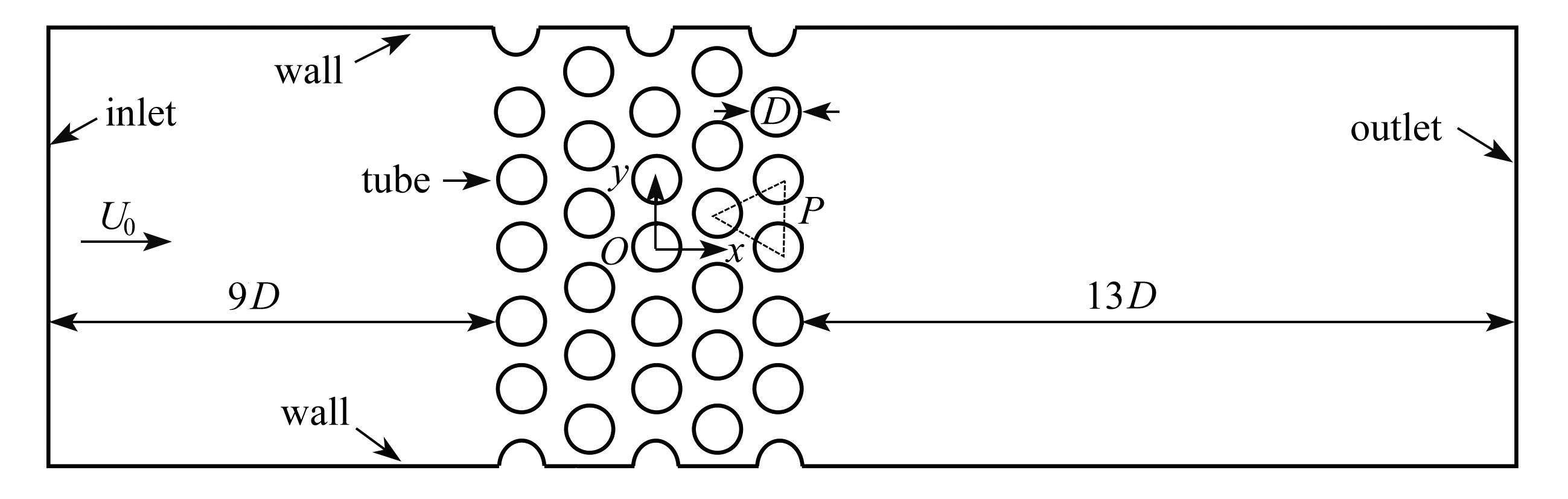Fig.1 Flow field geometry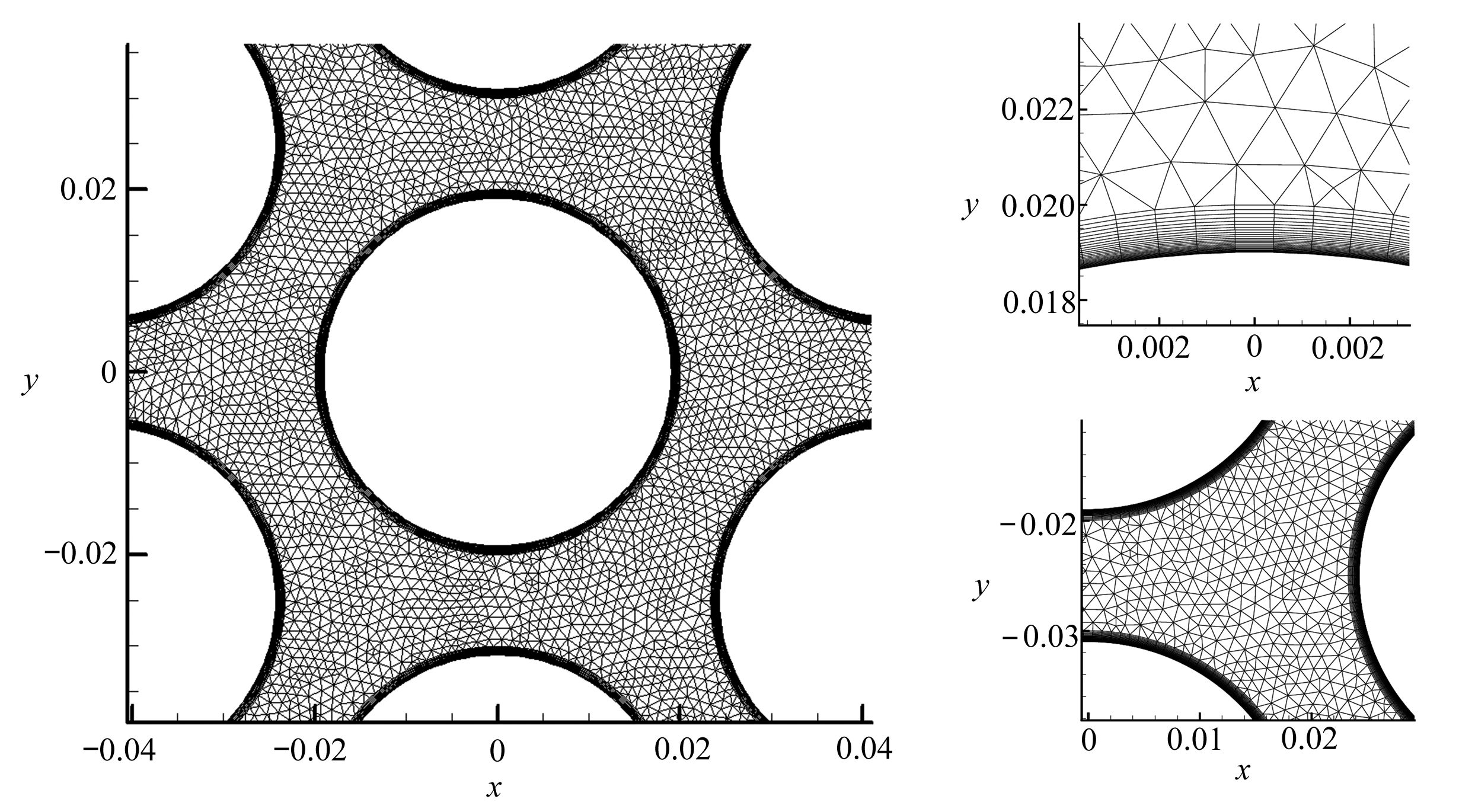Fig.2 boundary layer and flow channel mesh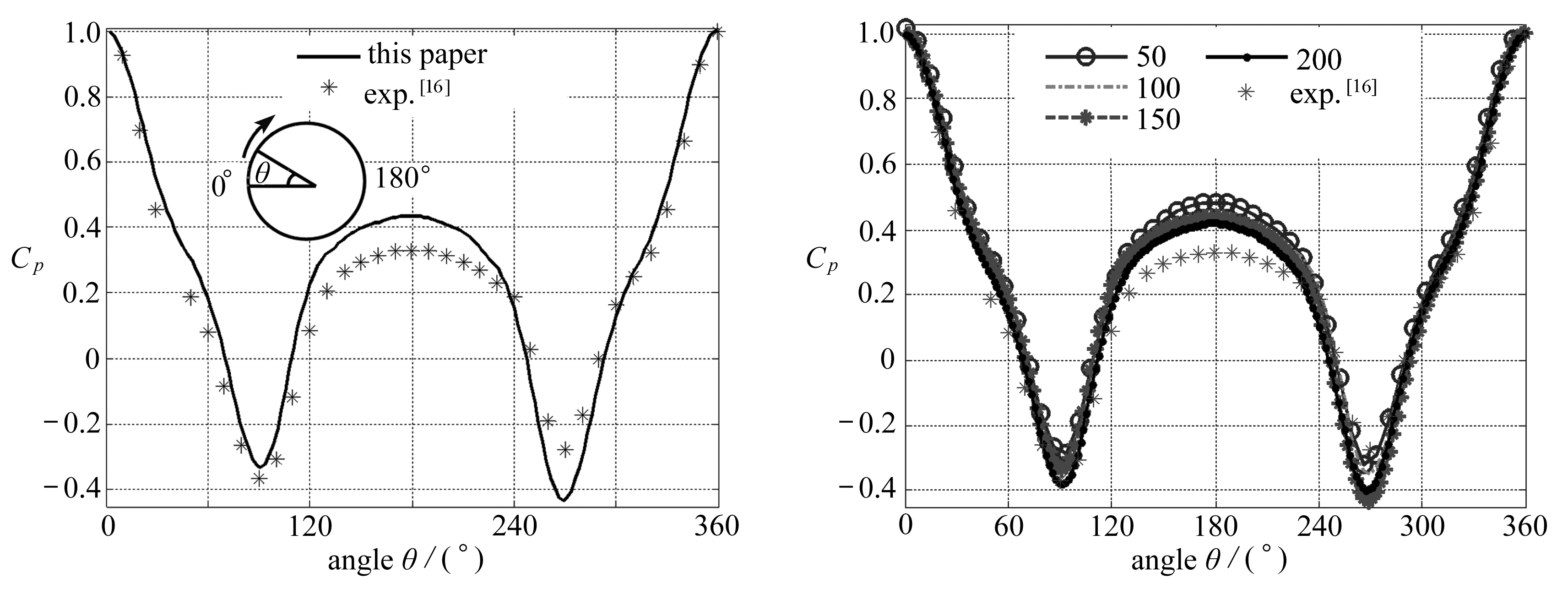Fig.3 U0=7 m/s, pressure distribution Fig.4 Mesh-independent calculation results
on the central tube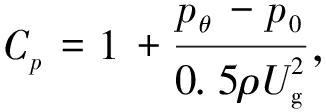(1)

### 1.2 流致振动模拟方法Fig.5 The flow-induced vibration model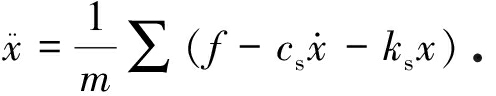(2)

## 2 计 算 结 果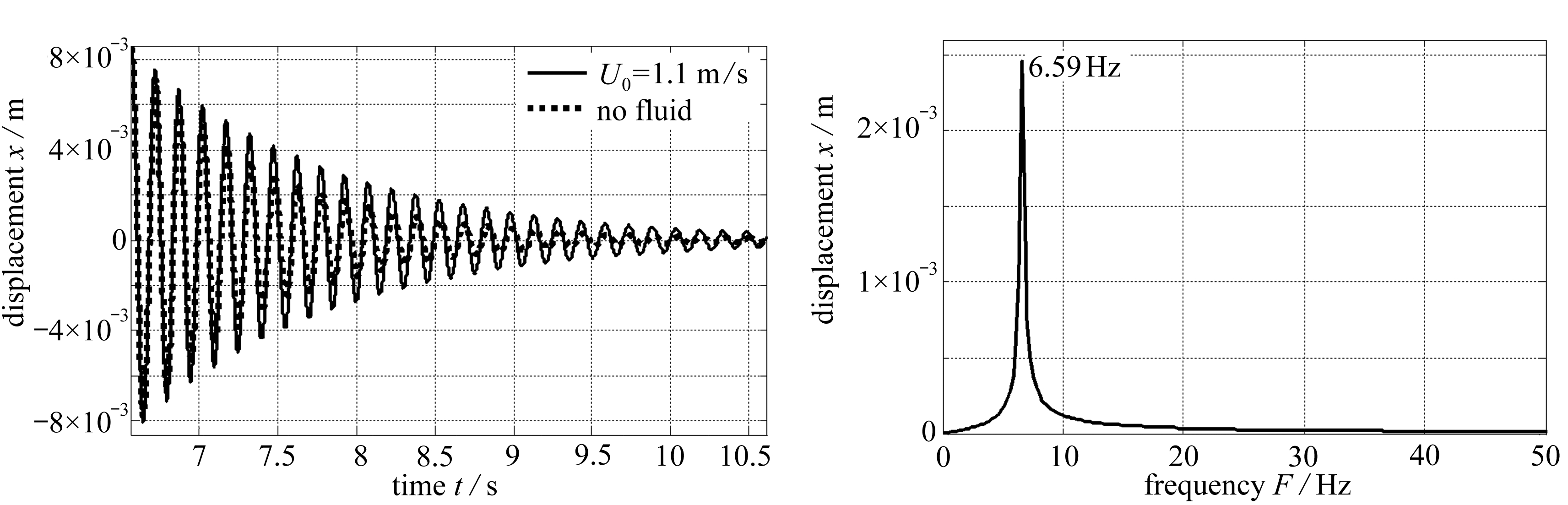Fig.6 The displacement time series for U0=1.1 m/s Fig.7 The displacement spectrum for U0=1.1 m/s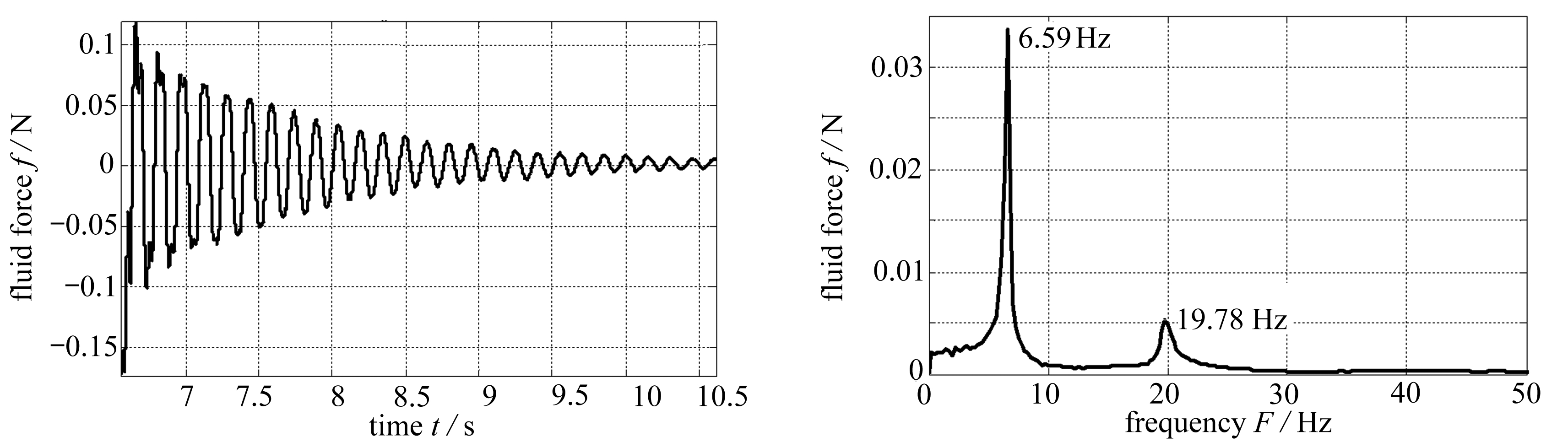Fig.8 The fluid force time series for U0=1.1 m/s Fig.9 The fluid force time series for U0=1.1 m/s

## 3 流体力模型研究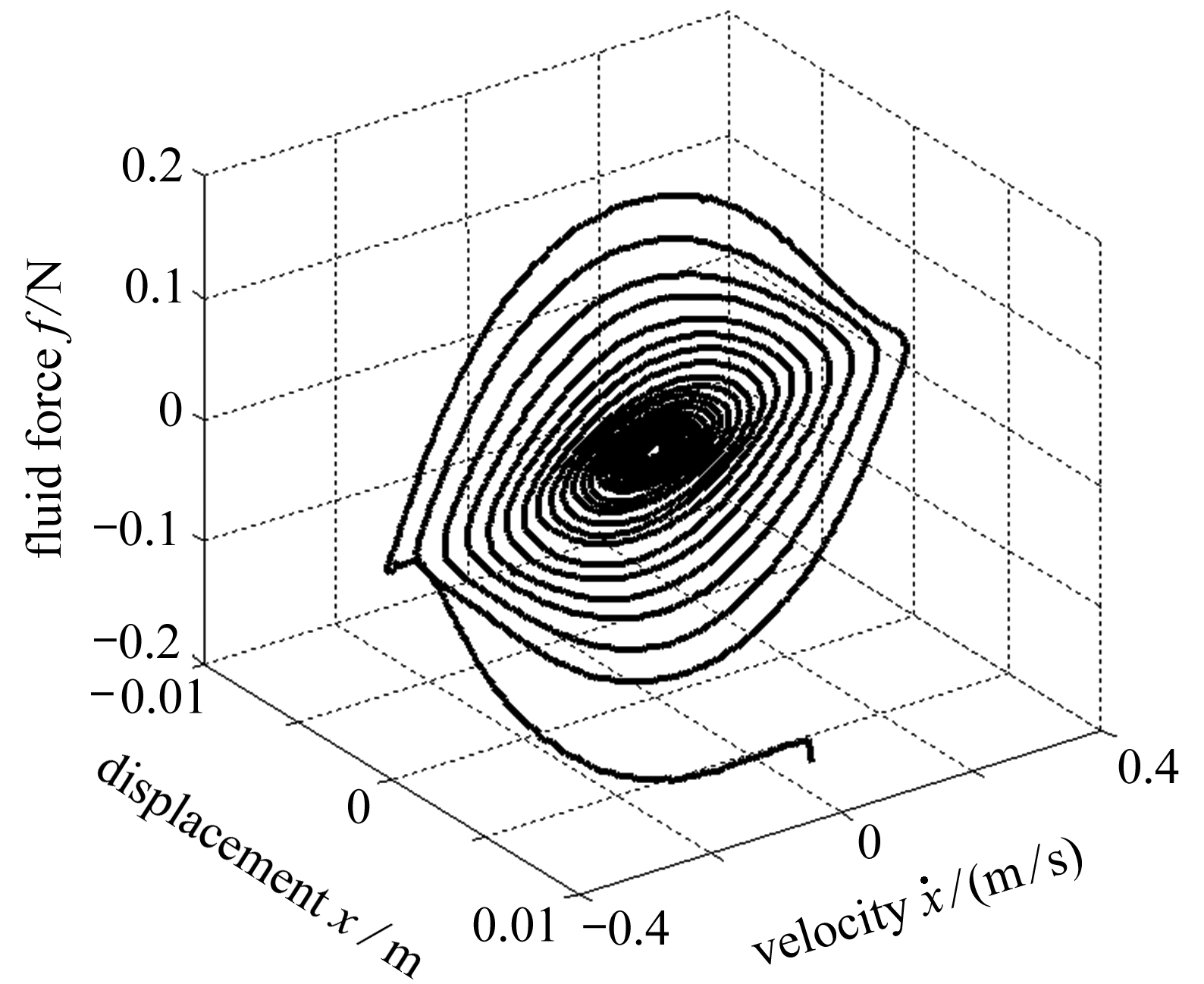(a) 力-状态图
(a) The force-state map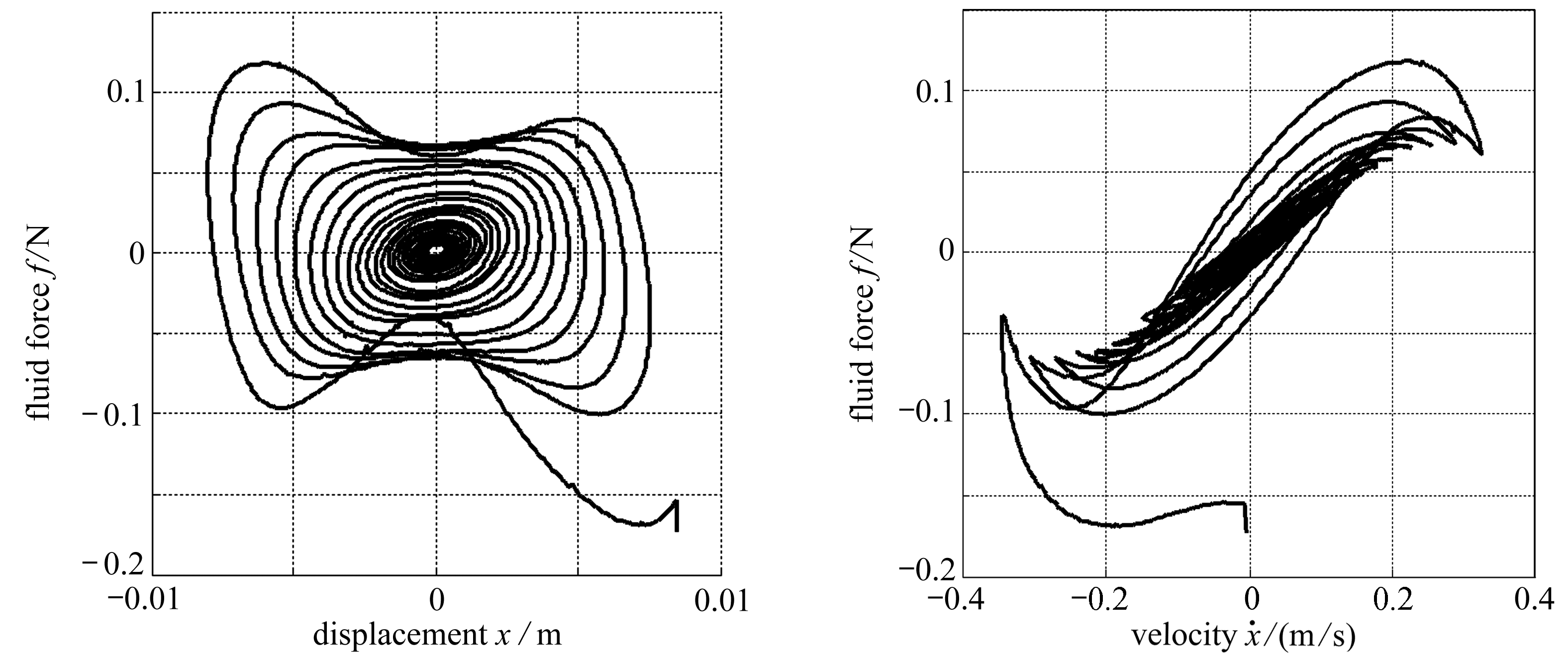(b) 力-位移图 (c) 力-速度图
(b) The force-displacement map (c) The force-velocity map

Fig.10 Force-state map and its projections(U0=1.1 m/s)(3)(4)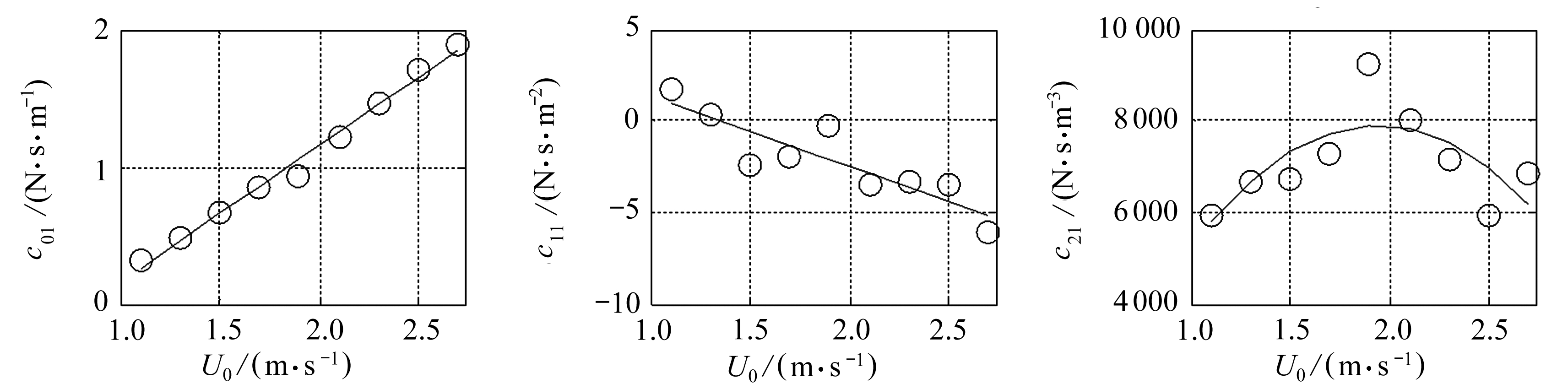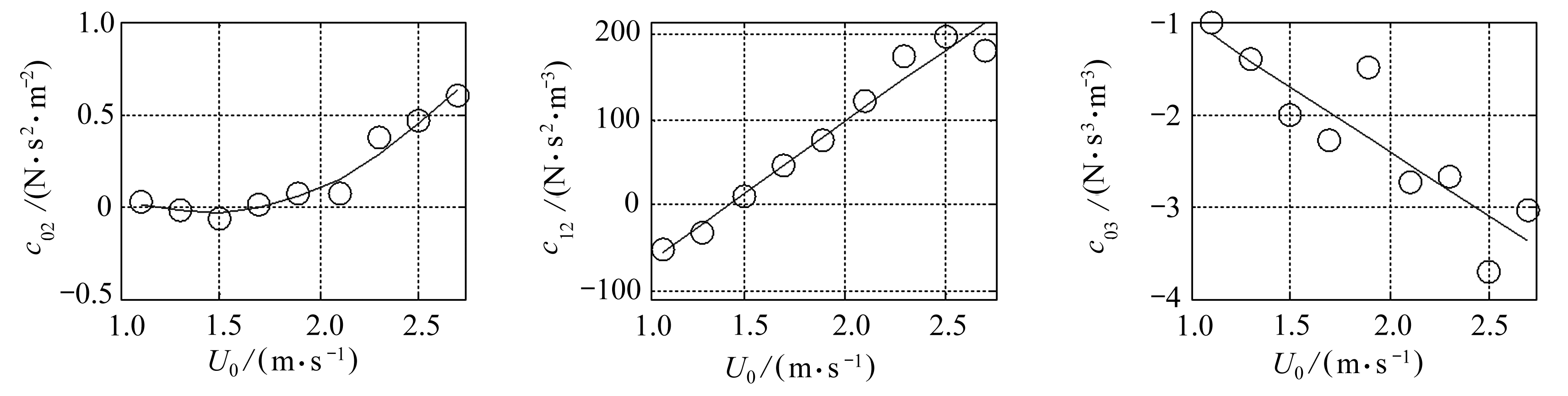Fig.11 Fluid force coefficients

Table 1 Fluid force coefficients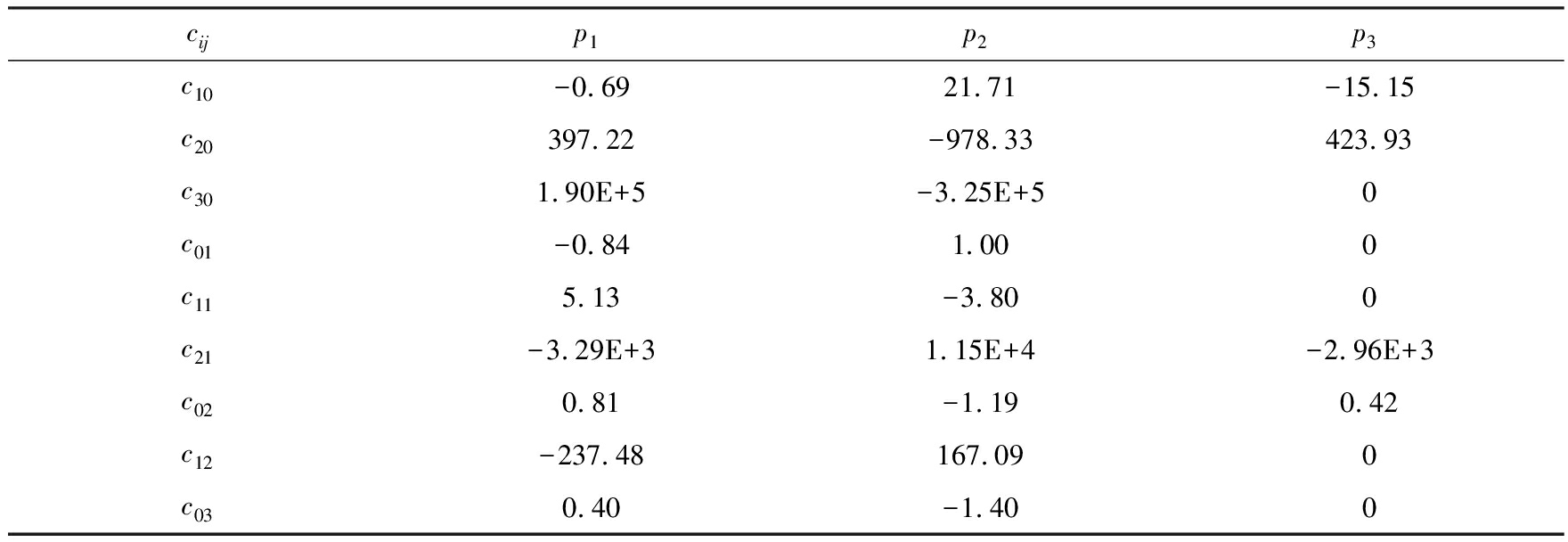cijp1p2p3c10-0.6921.71-15.15c20397.22-978.33423.93c301.90E+5-3.25E+50c01-0.841.000c115.13-3.800c21-3.29E+31.15E+4-2.96E+3c020.81-1.190.42c12-237.48167.090c030.40-1.400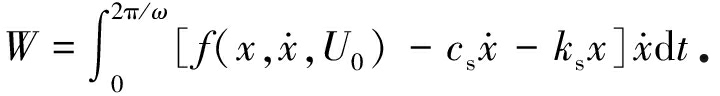(5)

x=Asin(ωt)

(6)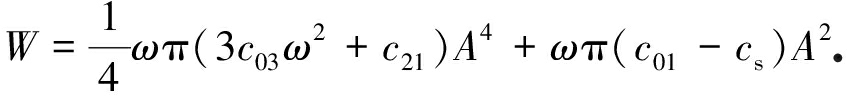(7)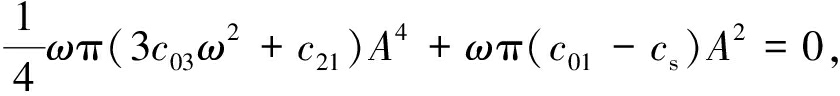(8)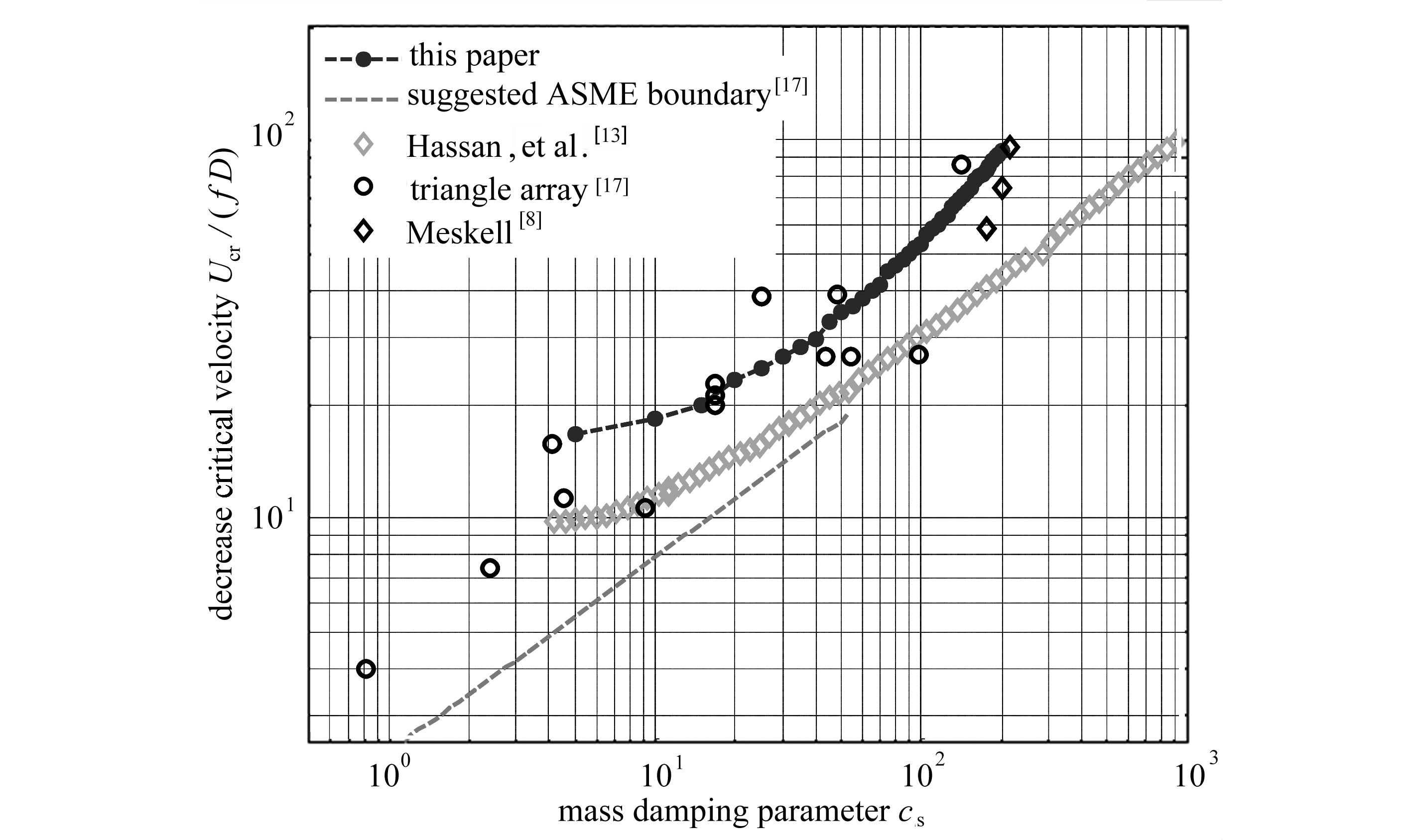Fig.12 Comparison of the predicted critical flow velocity with the experimental data

## 4 结 论

 AU-YANG M K.Flow-Induced Vibration of Power and Process Plant Components: a Practical Workbook[M].New York: ASME Press, 2001.

 LEVER J H.A theoretical model for fluid-elastic instability in heat exchanger tube bundles[D].PhD Thesis.Hamilton: McMaster University, 1983.

 聂清德, 郭宝玉, 丁学仁, 等.换热器管束中的流体弹性不稳定性[J].力学学报, 1996, 28(2): 151-158.(NIE Qingde, GUO Baoyu, DING Xueren, et al.Fluidelastic instability of heat exchanger array[J].Chinese Journal of Theoretical and Applied Mechanics, 1996, 28(2): 151-158.(in Chinese))

 PRICE S J, PAIDOUSSIS M P.An improved mathematical model for the stability of cylinder rows subject to cross-flow[J].Journal of Sound and Vibration, 1984, 97(4): 615-640.

 PRICE S J, VALERIO N R.A non-linear investigation of single-degree-of-freedom instability in cylinder arrays subject to cross-flow[J].Journal of Sound and Vibration, 1990, 137(3): 419-432.

 蒋天泽, 李朋洲, 马建中.两相横流作用下的旋转三角形管束流弹失稳研究[J].核动力工程, 2019, 39(1): 33-36.(JIANG Tianze, LI Pengzhou, MA Jianzhong.Fluidelastic instability of rotated triangle tube bundles subject to two-phase cross-flow[J].Nuclear Power Engineering, 2019, 39(1): 33-36.(in Chinese)).

 CHEN S S, JENDRZEJCZYK J A.Stability of tube arrays in crossflow[J].Nuclear Engineering & Design, 1983, 75(3): 351-373.

 MESKELL C.Identification of a non-linear model for fluidelastic instability in a normal triangular tube array[D].PhD Thesis.Dublin: Trinity College, 1999.

 MASRI S F, CAUGHEY T K.A nonparametric identification technique for nonlinear dynamic problems[J].Journal of Applied Mechanics, 1979, 46(2): 433-447.

 冯志鹏, 张毅雄, 臧峰刚.直管束流固耦合振动的数值模拟[J].应用数学和力学, 2013, 34(11): 1165-1172.(FENG Zhipeng, ZHANG Yixiong, ZANG Fenggang, Numerical simulation of fluid-structure interaction for tube bundles[J].Applied Mathematics and Mechanics, 2013, 34(11): 1165-1172.(in Chinese))

 吴皓, 谭蔚, 聂清德.正方形排布管束流体弹性不稳定性数值计算模型研究[J].振动与冲击, 2013, 32(21): 102-106.(WU Hao, TAN Wei, NIE Qingde.A numerical model for fluid elastic instability of a square tube array[J].Journal of Vibration and Shock, 2013, 32(21): 102-106.(in Chinese))

 GILLEN S, MESKELL C.Numerical analysis of fluidelastic instability in a normal triangular tube array[C]//ASME Pressure Vessels & Piping Conference.Prague, Czech Republic, 2009.

 HASSAN M, GERBER A, OMAR H.Numerical estimation of fluidelastic instability in tube arrays[J].Journal of Pressure Vessel Technology, 2010, 132(4): 041307.

 ROMBERG O, POPP K.Fluid-damping controlled instability in tube bundles subjected to air cross-flow[J].Flow, Turbulence and Combustion, 1998, 61: 285-300.

 CHEN S S.圆柱结构的流动诱发振动[M].冯振宇, 张希农, 译.北京: 石油工业出版社, 1993.(CHEN S S.Flow-Induced Vibration of Circular Cylindrical Structures[M].FENG Zhenyu, ZHANG Xinong, transl.Beijing: Petroleum Industry Press, 1993.(Chinese version))

 MAHON J, MESKELL C.Surface pressure distribution survey in normal triangular tube arrays[J].Journal of Fluids and Structures, 2009, 25(8): 1348-1368.

 KANEKO S, NAKAMURA T, INADA F, et al.Flow-Induced Vibrations Classifications and Lessons From Practical Experiences[M].Academic Press, 2014.

# NumericalStudyofFluidelasticInstabilityFluidForceModelforNormal-TriangleTubeArrays

LIU Jian, ZHANG Yixiong, FENG Zhipeng, YE Xianhui, CHEN Guo, QI Huanhuan

(Science and Technology on Reactor System Design Technology Laboratory, Nuclear Power Institute of China,Chengdu 610213, P.R.China)

Abstract: To study the fluidelastic instability (FEI) fluid force model, the FEI process controlled by the fluid damping mechanism was taken as the research object, and the fluid-tube interaction was simulated with one elastic tube in the middle of a normal-triangle tube array under a series of free stream flow velocities.A polynomial function was chosen as the fluid force model, the simulated fluid forces and the tube displacements were used to calculate the unknown coefficients in the fluid force model.The influence of the flow velocity on the fluid force model was introduced and the relationship between fluid force coefficients and inlet flow velocities was fit.A fluid force model related to tube displacements, tube velocities and flow velocities was obtained.The predicted FEI critical flow velocities with the proposed fluid force model were compared to the experimental data and numerical results given by literatures.The fluid force model, constructed with the proposed method based on numerical results and the given mathematical function, can capture the main characteristics in the flow tube array interaction, and reasonably predict the FEI critical velocity.

Key words: tube array; flow-induced vibration; computational fluid dynamics; fluid force model; fluidelastic instability

*收稿日期： 2019-09-12；

ⓒ 应用数学和力学编委会，ISSN 1000-0887

http://www.applmathmech.cn

DOI: 10.21656/1000-0887.400269

Foundation item: The National Natural Science Foundation of China(51606180;11872060)